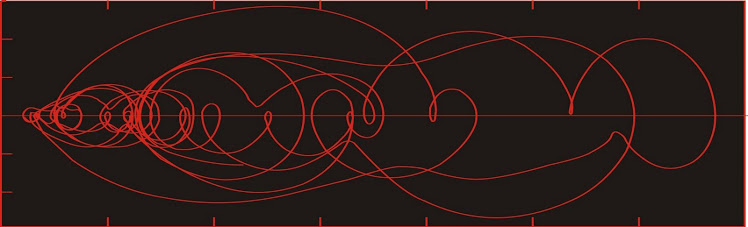## Dust flux, Vostok ice coreTwo dimensional phase space reconstruction of dust flux from the Vostok core over the period 186-4 ka using the time derivative method. Dust flux on the x-axis, rate of change is on the y-axis. From Gipp (2001).

## Friday, June 17, 2011

### Information theoretic approaches to characterizing complex systems, part 2: complexity of climate proxy data from probability density plots in state space

Last time the World Complex applied simple information theory to the probabilities of particular state transitions within the constructed epsilon machine representations of paleoclimate proxies.

Probability density is calculated by the method outlined here, and below, we see one frame of data for the oceanographic condition proxy (mid-ocean d13-C variability). In essence, the data are unfolded into two dimensions using the time delay method of phase space reconstruction. The probability density is estimated by mapping out a set of overlapping boxes through phase space, and the amount of time the reconstructed state lies within each box is calculated over a window (I have used 270-thousand-year windows--your mileage may vary). Similar windows have been created at 30 thousand year intervals going back nearly two million years.

The entropy of this distribution of data is calculated by reducing the number in each cell in the array above to a probability (a fraction of 270 thousand year duration). In the calculation of the above array, each observation is slotted into four separate cells, so the total "probabilities" add up to 4. The ideal correction would be to count up one cell in every four. What works as well is to use p(i) = t(i)/270,000, where t(i) are the elements of the array, sum up [-Σp(i)log p(i)]  for all non-zero terms and divide the total by four.

Calculating entropy for all windows for the oceanographic condition proxy and plotting the results below . . .

Higher values indicate higher entropy, which we normally equate with greater complexity. Oceanographic changes were highly complex in the early Quaternary (until about 1150 thousand years ago); but complexity fell through the middle Quaternary, reaching a minimum at about 750 thousand years ago); increased until
about 450 thousand years ago, and has generally declined since.

I should point out here that when we average data over a window (say from 0-270 thousand years ago), the standard is to plot the result at the midpoint of the window (i.e., 135 thousand years ago). When you look at a plot of the 200-day moving average for a stock price, they plot that average at the end of the window, rather than the middle. This is an important difference.

If we consider individual probability density plots, we see that the area of phase space occupied by the attractor over the Quaternary has varied, but is generally about the same size at different times. We shall see below that this is not the case for the ice volume proxy and the paleomonsoon strength proxy.

The variation of complexity in the ice volume proxy phase space reconstruction with time is as follows:

There is a marked increase in complexity from the Early Quaternary (right side of the graph) to the Late Quaternary (left side of the graph). That complexity has dropped rather sharply in the most recent windows, and this last bit of variability appears to exceed the variability within the graph. Is this an indication we are on the cusp or a major change to the global climate system?

The variation in complexity for the paleomonsoon proxy over the past 2 million years is as follows:

Once again there is a significant increase in complexity in the behaviour of the phase space portrait of the paleomonsoon strength proxy throughout the Quaternary. In the early Quarternary the behaviour of the system is quite simple, and is dominated by tight orbits around a single area of high probability. Complexity is much higher in the late Quaternary, but does fall off somewhat fairly recently.

Probability density plot for phase space portrait of paleomonsoon strength proxy,
over a window from 1421 to 1691 thousand years ago.

Probability density plot for phase space portrait of paleomonsoon strength proxy,
from 281 to 551 thousand years ago. The larger number of LSA areas (high probability)
suggests greater complexity of behaviour in the Late Quaternary.

The overall picture of complexity through the Quaternary is in accordance with that inferred from the epsilon machine computation of our last installment.